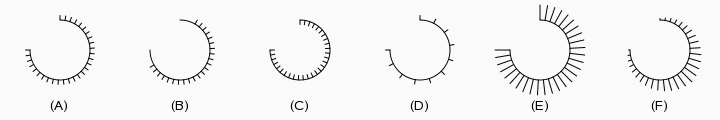# OEDrawEyelashSurfaceArc¶

bool OEDrawEyelashSurfaceArc(OEDepict::OEImageBase &image,
const OEDepict::OE2DPoint &center,
double bgnAngle, double endAngle, double radius,
const OEDepict::OEPen &pen, double edgeAngle=10.0,
unsigned int patternDirection=OEPatternDirection::Outside,
double patternAngle=10.0,
double minPatternWidthRatio=0.15,
double maxPatternWidthRatio=0.15)


Draws an arc with the OESurfaceArcStyle_Eyelash style.OEDrawEyelashSurfaceArc

image
The image on which the arc is drawn.
center
The center of the arc.
bgnAngle, endAngle
The two endpoints of the arc. Both angles are in degrees and their values have to be in a range of $$[0.0^{\circ} - 360.0^{\circ}]$$. Angles are interpreted such that $$0.0^{\circ}$$ and $$360.0^{\circ}$$ degrees are at the 12 o’clock position, $$90.0^{\circ}$$ degrees corresponds to 3 o’clock, etc.
pen
The graphical properties of the arc.
edgeAngle

The angle (in degrees) between the end points of the arc and the points where the pattern of the arc starts.

See example (B) in Figure: OEDrawEyelashSurfaceArc.

patternDirection

Specifies whether the pattern is drawn inside or outside of the arc. This value has to be from the OEPatternDirection namespace.

See example (C) in Figure: OEDrawEyelashSurfaceArc.

patternAngle

Specifies the angle (in degrees) between the patterns of the arc. The angle has to be in a range of $$[1.0^{\circ} - 90.0^{\circ}]$$

See example (D) in Figure: OEDrawEyelashSurfaceArc.

minPatternWidthRatio, maxPatternWidthRatio

Specifies the width of the pattern of the arc relative to its radius. The min ratio is applied to the end of the arc while the max ratio specifies the width of the patterns at the middle of the arc. Both ratios have to be in a range of $$[0.01 - 1.00]$$.

See examples (E) and (F) in Figure: OEDrawEyelashSurfaceArc.